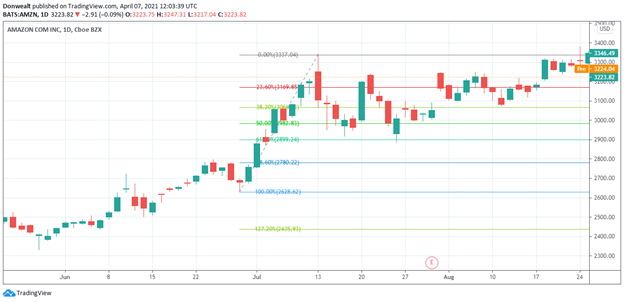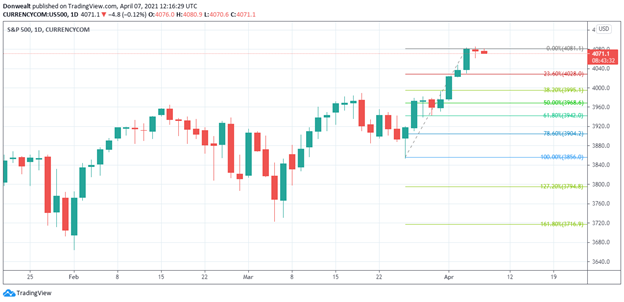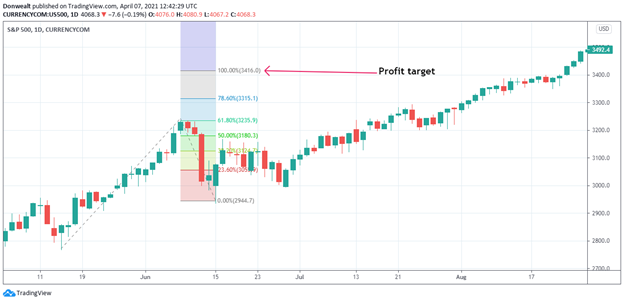Since 2013

• 100% Quantified, data-driven and Backtested
• We always show our results!
• Signals every day via our site or email
• Cancel at any time!

# What Does Fibonacci Mean In Trading?

Last Updated on 11 September, 2023 by Samuelsson

Fibonacci is a common term that almost every Forex or stock trader is acquainted with. If you are a trader, you must have heard stock market analysts on TV channels suggesting that the stock is trading around some ‘Fibonacci’ levels. But what does this Fibonacci mean in trading?

In trading, the Fibonacci retracement tool, which is the most common of all the Fibonacci tools, is an important technical analysis tool that uses horizontal lines to indicate where support and resistance are likely to occur in the future. The retracement levels are calculated using the Fibonacci number sequence, and they are usually expressed as a percentage of the preceding price swing, with the common levels being 61.8%, 38.2%, and 23.6% retracement levels. You can use these levels to determine your entry point, as well as your stop loss and profit targets.

In this article, we will be looking at everything you need to know about the Fibonacci tool. Here are the things we would cover:

• Fibonacci basics: what you should know about the Fibonacci tools
• Why is Fibonacci used in trading?
• How does Fibonacci work in trading?
• How accurate is Fibonacci retracement?

## Fibonacci basics: what you should know about the Fibonacci tools

In trading, Fibonacci refers to a set of technical analysis tools, such as the Fibonacci retracement/extension tool, Fibonacci expansion tool, Fibonacci arcs, and Fibonacci fan, which use unique ratios derived from the Fibonacci number series to identify special price levels and periods that may have an impact on the price movement.

One interesting thing about the Fibonacci tools is that they lead the price, as they identify potentially important price levels and periods before the price gets there. Interestingly, the tools are available on every charting platform. To understand how these tools came about, let’s take a look at the origin of the Fibonacci numbers.

### The origin of the Fibonacci number series

The origin of the Fibonacci number series can be traced back to the 13th century when an Italian mathematician, Leonardo Fibonacci, described a unique sequence of numbers in his book, “Liber Abaci (Book of Calculation)”. He described a number sequence in which each number in the series — except 0 and 1 — is the sum of the two numbers before it.

Thus, the number sequence looks like this: 0, 1, 1, 2, 3, 5, 8, 13, 21, 34, 55, 89, 144, 233, 377, 610, 987, 1597, and continues like that till infinity. Taking a closer look at the numbers, one thing you will notice is that from 21 and above, each number is about 161.8% of the number immediately before it or 61.8% of the number immediately after it. For example, if you divide 34 by 55, you will get 0.618, meaning that 34 is 61.8% of 55. Going the other way round, if you divide 55 by 34, you will get 1.618, which is widely regarded as the golden ratio — denoted in mathematics with the Greek letter φ — because of its occurrence in several aspects of nature.

The golden ratio (1.618) and the inverse (0.618) are quite prevalent in different aspects of our natural world and human arts. These ratios have been observed in the spiral galaxies of outer space, tree branches, sunflowers, rose petals, human faces, Leonardo da Vinci’s Mona Lisa, the Parthenon, and the ancient Greek vases, as well as in the nautilus shell, which spirals at about the same level as the percentages from the golden ratio and its inverse.

### How the Fibonacci ratios and levels are derived

As we stated earlier, dividing a number in a number in the sequence with the number immediately before it gives the golden ratio — 1.618 — and the inverse is 0.618. These ratios can be expressed as percentages: 161.8% and 61.8%. Aside from the golden ratio and its inverse, other ratios can be derived from the numbers in the Fibonacci sequence.

For instance, dividing a number by the number two places to the right — say, 89 divided by 233 — would give 0.382 (38.2%), while the inverse would give 2.618 or 261.8%. Furthermore, if you a number in the sequence by a number three places after it — say, 34 divided by 144 — you will get the ratio 0.236 (23.6%).

Some do not consider the 0.50 (50%) and 1.00 (100%) Fibonacci ratios. But 1, 1, and 2 are members of the sequence and dividing 1 by 2 gives 0.50 (50%), while dividing 1 by 1 gives 1.00 (100%).

In technical analysis, these ratios/percentages are used to estimate how far the price can retrace after a swing (retracement levels) and how far the price can expand in the next swing (expansion levels). When using the Fibonacci retracement tool, the ratios/percentages below 1.00 (100%), such as 23.6%, 38.2%, and 61.8%, are considered retracement levels, where the price can retrace to after an impulse swing before starting another impulse swing. On the other hand, in the Fibonacci expansion tool, all the ratios/percentages below and above 100% can show expansion levels where the next price swing can get to from where the pullback ended.

### The common Fibonacci tools: Fibonacci retracement/extension and Fibonacci expansion

#### Fibonacci retracement/extension tool

When attached to the price chart, the Fibonacci retracement tool draws horizontal lines at 23.6%, 38.2%, 50%, and 61.8% of the preceding price swing. These are retracement levels where a pullback may reverse to start a new impulse swing in the trend direction. You can use these levels in your trading strategies to determine your entry point and stop loss levels.The Fibonacci retracement tool can also be set to show some extension levels beyond the 100% retracement levels.

#### Fibonacci expansion tool

In some trading platforms, such as the MT4, MT5, and TradingView, there is a separate expansion tool that shows the price expansion levels. This tool traces the pullback swing and uses the size of the pullback to estimate how far the next impulse swing can expand in the trend direction. The expansion levels can be used to estimate profit targets.## Why is Fibonacci used in trading?

From our discussion so far, you can deduce that the Fibonacci retracement and expansion tools can be used to predict potential support and resistance levels in the market. These tools show these levels before the market gets to such levels, so they lead the price, which is a very remarkable property because traders want to know what will happen ahead of time so that they can plan what to do.

So, Fibonacci is used in trading because it helps traders to analyze the market and plan for their trades ahead of time. Getting to know these important areas would help a trader to decide when to open or close a trading position, as well as where to place stops and limits to their trades.

## How does Fibonacci work in trading?

When properly attached to the price chart, the Fibonacci tools work by identifying possible levels where the price may reverse. Since the price may reverse from those levels, they are regarded as areas of support and resistance. The Fibonacci tools identify these levels by drawing horizontal lines at certain percentages of the preceding price swing where the price might retrace to before reversing — in the case of the Fibonacci retracement levels — or in the case of the expansion/extension levels, where the next impulse swing might get.

So, for the retracement tool, the idea is to identify where the recent pullback might reverse to start a new impulse swing (a price swing in the trend direction). But to use this tool, you have to properly attach it to the right price swing. Here is how to do it:

• Identify the trend direction
• Spot the most recent impulse swing when the price has started pulling back
• Pick the Fibonacci retracement tool on your charting platform and attach it to that most recent impulse swing, starting from the beginning of that swing. That is, if you are dealing with an upswing in an uptrend, you start from the low to the high, but if you are dealing with a downswing in a downtrend, you start from the high to the low.
• Once attached, the tool will display horizontal lines marking the percentage retracement of that impulse swing, such that the beginning of that impulse swing would bear a 100% retracement level.## How accurate is Fibonacci retracement?

Now, you may be wondering how accurate Fibonacci retracement is and probably asking the question: “Is Fibonacci trading good?” Well, Fibonacci trading is fine for those who know how to make good use of the tool. The retracement levels can help traders identify potential areas to watch out for trade setups or place their stop loss orders.

Overall, Fibonacci retracement tends to show a higher level of accuracy when used in a longer timeframe and can become even more powerful when used in conjunction with other indicators or technical signals. However, like everything in trading, the Fibonacci retracement levels can never be 100% accurate in identifying potential reversal levels.

It is not all the time that the price respects the Fibonacci levels. And even when the price reverses around a Fibonacci retracement level, it must have broken the levels before it before reaching the one it reversed at. For instance, to reach the 61.8% level, the price must have broken the 23.6% and 38.2% levels. So, there is no way of knowing at which level it would reverse.

Fibonacci retracement is just a single tool and should be used alongside other indicators, such as trend lines, volume, moving average convergence divergence (MACD), and moving averages, to increase the chances of getting high probability trade setups. Normally, the Fibonacci levels are best used to trade a trending market, so you need those tools that can help you to identify the trend direction. Additionally, when using the Fibonacci levels to make a trade entry decision, you need a good trade trigger, such as reversal candlestick patterns or oscillator overbought/oversold signals.

By and large, these are the three ways you can use the Fibonacci levels in your trading:

• Trade entry: You can use the Fibonacci retracements levels to identify your trade entry because they provide some areas of interest to watch when the price is making a pullback. A good entry should show a reversal candlestick pattern, such as the pin bar or engulfing pattern, ending a pullback to a Fibonacci level (say 61.8%) in a trending market. Some traders may also place limit orders at Fibonacci levels, but this practice is risky.
• Stop loss level: Some traders use Fibonacci retracement levels to know where to place their stop loss orders. A common practice is to place the stop loss order beyond the 100% retracement level if the trade entry is around the 50% or 60% retracement level.
• Profit target level: Traders use the Fibonacci expansion levels, especially the 100% expansion level, to estimate their profit targets. Those who like taking partial profits at multiple levels can use the 100%, 161.8%, and 261.8% expansion levels for their profit targets.